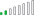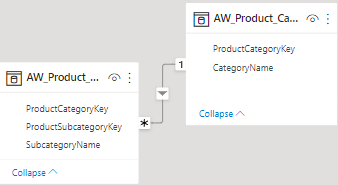cancel
Showing results for
Did you mean:Helper I

## Multiply values in columns (Different tables)

Say I have one table like this:

 a 1,02 b -0,4 c -5 a -3,2 b 3 c -4,5

And the other table like this:

 a 839403284 b 932848032 c 39842048

How to I multiply each of the values in the first table with the corresponding values in the second table?

And what should the relation on the "Model"-page look like?

Thanks!

1 ACCEPTED SOLUTIONCommunity Support

Hi @sigridr ,

Please refer to my pbix file to see if it helps you.

Create a measure.

``Measure = MAX('Table'[value])*MAX('Table (2)'[val])``Best Regards

Community Support Team _ Polly

If this post helps, then please consider Accept it as the solution to help the other members find it more quickly.

18 REPLIES 18Community Support

Hi @sigridr ,

Please refer to my pbix file to see if it helps you.

Create a measure.

``Measure = MAX('Table'[value])*MAX('Table (2)'[val])``Best Regards

Community Support Team _ Polly

If this post helps, then please consider Accept it as the solution to help the other members find it more quickly.Super User

Hi @sigridr
My Undestanding that you cannot create calculated columns and you cannot create or edit relationships. Then try to create New Measure

``````NewMeasure =
SUMX (
Table1,
VAR Name = Table1[Name]
VAR Value1 = Table1[Value]
VAR Value2 =
MAXX ( FILTER ( Table2, Table2[Name] = Name ), Table2[Value] )
RETURN
Value1 * Value2
)``````Frequent Visitor

Hello, in the idea you provided, what is the "name"? i understand what table and values are but i dont understand whats supposed to go where is says nameSuper User

The name of the first column that contains "a", "b", "c"Frequent Visitor

This worked perfectly for me thank you very much!Helper I

This works for most categories:But for certain others it's completely wrong... why would that be?Frequent Visitor

unrelated, but this solved a problem I was stuck in for days. thank you.Super User

can you share a picture of the diagram view showing the relationship between the tables ?Helper I

It's a simple, many-to-one relationship.
One table has only one occurence of each value, while the other table has lots of occurences of each value. Does it matter wheter it's formatted as numbers or text? (the values that are related are 4-number values)Super User

It doesn't matter whether they are numbers or text but they both need to be the same. and if its text you need to make sure that there are no leading or trailing spaces in either column, as that would throw the relationship outHelper I

All of that should be in order.Resolver II

Firstly you can create a relationship between the two tables based on the common column. Now you can use the below DAX to do the multiplication -

`Multilple = TABLE1[COLUMN1]* RELATED(TABLE2[COLUMN2])`
Model Should look something like this, just a sample image -Let me know this helped you.Helper I

It won't let me chose the right columns.. 😞
What should I do?Resolver II

Create a relationship between the two tables first.Helper I

That should be in order, but there are still some of the calculations that are completely wrong..Resolver II

Firstly you can create a relationship between the two tables based on the common column. Now you can use the below DAX to do the multiplication -

`Multiply = TABLE1[COLUMN1]* RELATED(TABLE2[COLUMN2])`
Model Should look something like this, just a sample image -Let me know if this helped you.Super User

If you know that there will only be 1 entry per item in the 2nd table then you can create a one-to-many relationship between the tables. You would then be able to use the RELATED function to do the multiplication. e.g. you could create a column on the first table like

``Multiplied value = 'Table 1'[Value] * RELATED('Table 2'[Value])``Helper I

It won't let me make that formula.. 😕